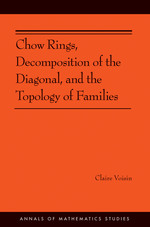# Chow Rings, Decomposition of the Diagonal, and the Topology of Families

Claire Voisin
Pages: 176
https://www.jstor.org/stable/j.ctt5hhp7w

1. Front Matter
(pp. i-iv)
(pp. v-vi)
3. Preface
(pp. vii-viii)
Claire Voisin
4. Chapter One Introduction
(pp. 1-14)

These lectures are devoted to the interplay between cohomology and Chow groups, and also to the consequences, for the topology of a family of smooth projective varieties, of statements concerning Chow groups of the general or very general fiber.

A crucial notion is that of theconiveauof a cohomology. A Betti cohomology class has geometric coniveau ≥cif it is supported on a closed algebraic subset of codimension ≥c. The coniveau of a class of degreekis$\le \frac{k}{2}$. As a smooth projective varietyXhas nonzero cohomology in degrees 0, 2, 4, … obtained by...

5. Chapter Two Review of Hodge theory and algebraic cycles
(pp. 15-35)

We follow  and [101, II]. LetXbe a scheme over a fieldK(which in practice will always be a quasi-projective scheme, that is, a Zariski open set in a projective scheme). Let${{\cal Z}_{k}}(X)$be the group ofk-dimensional algebraic cycles ofX, that is, the free abelian group generated by the (reduced and irreducible) closedk-dimensional subvarieties ofXdefined overK. IfYXis a subscheme of dimension ≤k, we can associate a cycle$c(Y)\in {{\cal Z}_{k}}(X)$to it as follows: Set$c(Y)=\sum\limits_{W}{{{n}_{W}}}W, \caption {(2.1)}$where the sum is taken over thek-dimensional irreducible reduced components of...

6. Chapter Three Decomposition of the diagonal
(pp. 36-54)

The following result is essentially due to Bloch and Srinivas . Letf:XYbe a smooth projective morphism, whereXandYare smooth (for simplicity), and letZ∈ CHk(X) be a cycle inX. Consider the following property.

(∗)There exists a subvariety X′X such that for every yY, the cycle${{Z}_{y}}:={{Z}_{\left| {{X}_{y}} \right.}}:=j_{y}^{*}Z\in \text{C}{{\text{H}}^{k}}({{X}_{y}})$vanishes in$\text{C}{{\text{H}}^{k}}({{X}_{y}}-X_{y}^{\prime})$,where jyis the inclusion of the fiber Xy=f−1(y)into X.

Theorem 3.1.If Z satisfies the property(∗),then there exist an integer m> 0and a cycle Z′ supported in X′,...

7. Chapter Four Chow groups of large coniveau complete intersections
(pp. 55-87)

Consider a smooth complete intersection$X\subset {{\mathbb{P}}^{n}}$ofrhypersurfaces of degree${{d}_{1}}\le \cdots \le {{d}_{r}}$. By the Lefschetz hyperplane section theorem (see [101, II, 1.2.2]), the only interesting Hodge structure in the cohomology ofXis the Hodge structure on$H_{B}^{n-r}(X,\mathbb{Q})$, and in fact on the primitive part of it (that is, the orthogonal of the restriction of$H_{B}^{*}({{\mathbb{P}}^{n}},\mathbb{Q})$with respect to the intersection pairing). We will say thatXhas Hodge coniveaucif the Hodge structure on$H_{B}^{n-r}{{(X,\mathbb{Q})}_{\text{prim}}}$has coniveauc.

The Hodge coniveau of a complete intersection in projective space is computed as follows (see  for the...

8. Chapter Five On the Chow ring of K3 surfaces and hyper-Kähler manifolds
(pp. 88-122)

The following theorem is proved in .

Theorem 5.1 (Beauville and Voisin 2004).Let S be a K3surface, Di∈ CH1(S)be divisors on S and nijbe integers. Then if the0-cycle${{\sum }_{i,j}}{{n}_{ij}}{{D}_{i}}{{D}_{j}}\in \text{C}{{\text{H}}_{0}}(S)$is cohomologous to0on S, it is equal to0inCH0(S).

Proof. It suffices to prove that there is a 0-cycleoof degree 1, with the property that for any two line bundlesL,L′ onS,$L\cdot {L}'=\deg (L\cdot {L}')\ o\ \text{in}\quad \text{C}{{\text{H}}_{0}}(S). \caption {(5.1)}$

The cycleois defined to be the class of any point ofScontained in a (singular) rational curveCS. We claim...

9. Chapter Six Integral coefficients
(pp. 123-154)

Atiyah and Hirzebruch  found counterexamples to the Hodge conjecture (Conjecture 2.25) stated for degree 2k integralHodge classes (as opposed torationalHodge classes) whenk≥ 2. In degree 2, the most optimistic statements are true, due to the Lefschetz theorem on (1, 1)-classes.

In , Totaro revisited the examples of Atiyah and Hirzebruch and reformulated more directly the obstructions they had found, using the complex cobordism graded ring MU* (X) ofX. Let us first describe this ring that is defined for all differentiable compact manifolds: Given such anX, we consider first of all the objects...

10. Bibliography
(pp. 155-162)
11. Index
(pp. 163-163)